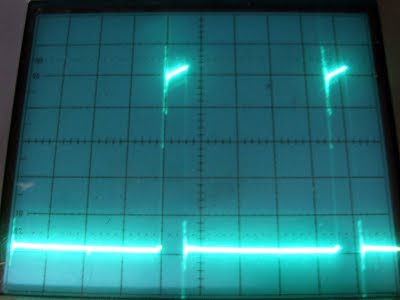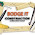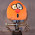## Thursday, 6 August 2009

### Motor Maths

In a previous post I showed the pitfalls of constant off time choppers like the A3977. Basically you have to set the off time long enough to be able to deliver the lowest current step of the microstepping, otherwise the steps are not equally spaced.

Forrest raised the point of how do you do that without a scope. It is fairly easy to calculate from the target current, supply voltage and motor resistance using nothing more complex than Ohm's law. It did take me a few days to come up with a formula that matched my measurements, but that was because I was accidentally running the chip without synchronous rectification enabled.

The motor current is equal to the reference voltage divided by 8 times the sense resistor. The maximum sense voltage is 0.5V, so a sensible value for the sense resistors is 0.2Ω, giving 2.5A maximum with 4V at the reference pin. My lash up uses two 0.5Ω resistors in parallel giving a 2A maximum.

The minimum current required on the first step of the microstep will be I × sin(π/2n), where n is the number of microsteps. In this case n is 8 so the smallest current step is 19.5% of the full current. To calculate the minimum off time needed we need to be able to work out what the duty cycle will be to get a given current.

Here is what the sense resistor waveform looks like when the current is set to 1A and the motor is stationary. The on period is 3μS and the off period is 20μS. The supply voltage is 12V.The sloping top of the waveform is actually an exponential curve, but at this scale it is very close to linear and to simplify the calculations I have just used the average value.

So we know that 1A flows from the supply for every 3 out of 23μS. That gives an average current from the supply of 1A × 3 /23 = 130mA. Indeed the supply current measures 260mA as there are two coils energised (I set it to full step mode to make this measurement).

When the chopper is on energy flows into the inductance of the motor, increasing its magnetic field slightly. During the off time the current flows in a loop consisting of the motor and the two low side transistors. Power is dissipated by the motor's resistance, so it loses energy by its magnetic field decreasing slightly. We can calculate the duty cycle by reasoning that the energy going in during the on state must equal the energy coming out in the off state.

The motor is a Lin 4118S-62-07 NEMA17 motor I got from Makerbot. It has a coil resistance of only 0.8Ω. That means the resistance of the sense resistor and the on resistance of the FETs in the chip are significant in the calculation.

During the on state current flows through one top transistor, the coil, one bottom transistor and the sense resistor. All the resistances convert electricity to heat so the power going into the magnetic field is the power drawn from the supply minus the resistive losses in the circuit.Power = VI or I2R, Energy = PT.

So we have (Vsupply × I - I2 × (Rmotor + Rsense + RDS(on) source +RDS(on) sink)) × Ton.

In this example (12 - (0.8 + 0.25 + 0.36 + 0.45)) * 3 = 30.4 μJ.

During the off state the current flows through the motor resistance and two low side transistors, so the energy lost is: I2 × (Rmotor + 2 × RDS(on) sink) × Toff.

In this example (0.8 + 2 × 0.36) × 20 = 30.4 μJ, so theory matches practice (using typical values from the datasheet for RDS(on)), always very satisfying.

So if we call the total resistance in the circuit with the switch on Ron and the total when it is off Roff we have: -
Ron = Rmotor + Rsense + RDS(on) source +RDS(on) sink = 1.86Ω
Roff = Rmotor + 2 × RDS(on) sink = 1.52Ω

Then Toff = Ton (V/I - Ron) / Roff
For our example if we set the minimum Ton (Tblank) to be 1μS, I = 0.195A, so Toff is 39μS.

At 1A Ton will then be ~6μS. So the minimum chopping frequency will be ~22kHz and the maximum will be 25kHz.

CT = Tblank / 1400 = 714pF, so use 680pF.
RT= Toff / CT =58K, so use 62K.

So in conclusion using the simple formulas above it is easy to calculate the correct values for a given motor, supply voltage and minimum current. I wish the datasheet and apps note had included this formula.

1.Slightly confused are you saying that for all Choping stepping controlers we need to adjust the chopping frequency to suit the motor used?
Or is this only applicable to the A3977 device or is the same for the A3980 A3982 A3983?

Thus the 298/298 device pair could also have the chop frequency adjusted to improve its efficentcy with a particular stepper motor?

2.This only applies to constant off time choppers with a minimum on time, which all of the above Allegro parts are.

L297 is constant frequency, which allows the mark space ratio to vary over a bigger range because both the mark and the space vary. It seems a better scheme to me, I don't know why constant off time is popular.

Having said that the L297 does not do micro stepping so you don't need the big range of currents. In my case I want to be able to set the current in the range 1-2A, but with micro stepping that is about a 10:1 range.

3.Also the L297/L298 is fast decay only, so less mark space ratio range is required, but it gives higher ripple current.

4.How did you arrive at 19.5% for the smallest step current?

5.Microstepping controls the current as a stepped sine wave. The minimum current required on the first step of the microstep will be I × sin(π/2n), where n is the number of microsteps. In this case n is 8 so the smallest current step is 19.5% of the full current.

6.Thanks for the reply....let me try to understand this formula...

Ex. my stepper motor has resistance 38.5 Ohm and 0.31A current

So i set my Allegro A3987 to limit max current to 0.3A. I am using 1/16 micro-step here.

minimum current = 0.3 x sin(pi/2x16)=0.000514 A, correct?

Or am i wrong (as always)....

7.Sorry, but yes you are wrong. You have calculated the sin with the angle in degrees rather than radians. sin(pi/32) is 0.098 so the current is 0.029A.

8.Nophead - Thank you for the quick reply and pointing out my mistake.

In this case the smallest step current is (0.029/0.3)x100=9.67% of the full current. Correct? If i were to look at the A3987 datasheet, under 1/16 step, the %Itrip(max) for step#2 is 9.38%. Am i looking in the right direction?

Actually, i am facing a problem with this A3987 in my project, that is, the step size is not even. Sometimes, the first step has a big jump. This is under no load condition and measured with a precise encoder attached to the shaft.

I am trying to adjust the fixed off-time as this is the only parameter that can be adjusted.

I tried to apply the formula shown in "Motor Math", i am not sure if what i did was correct, i need your help to verify:

Eg. Vmotor=24V

Rmotor = 38.5 x 2 (2 phases) = 77 Ohm
Ron = 78.55 Ohm (including Rds(on) source/sink)

Roff = 78.08 Ohm, Ton is fixed at 1us

Toff = Ton (V/I - Ron)/Roff = 1us(24/0.029 - 78.55)/78.08 = 9.59us

Frequency = 94.43kHz

The question is: do i really has to set Toff to 9.59us or do i follow the datasheet to set it to 30us typical? is this value critical?

Again, thanks a lot for your help!

9.I don't know why the datasheet current targets are slightly different from an exact sine wave but I would use those values. Sin (pi/32) is 9.8% but the chip uses 9.38%.

My formula gives the minimum off time to allow the lowest current step to be achieved. If you have the off time larger then the downside is lower frequency, which is only a problem if it becomes audible, and more ripple current. In my case, with a low inductance motor, the ripple current is very significant but might not be a problem with yours as I guess the inductance is much higher.

I looks like you are using a typical 12V unipolar motor with both coils in series. You might get better results using half of each coil. With both in series and a 24V supply you might not be able to get the 100% step as you are very close to the limit. I.e. your target current is virtually what the motor would draw connected to the supply and does not account for the voltage drops in the chip and the sense resistors and the off time.

Chopper drives only work well when the supply voltage is several times the motor voltage so you will get better performance in half coil mode, the inductance will be four times less so you get better high speed running.

If you steps are uneven it can be one of three problems: -

Off time too short, so cannot get the lowest current.

Off time too long so ripple current is large compared to the smallest current steps.

Supply voltage is too low for the specified current so you can't get the 100% current step.

I suspect you may have the third problem.

10.The motor is a Bipolar motor. I connected the 2 phases in series to get more torque. So 24V/77ohm = 0.31A which is the current rating of the motor and i wonder if the chopper drives can be activated properly and do it job.....

Looks like i may have to look for another motor with lower resistance.....

11.If it is a bipolar motor it will have 8 leads, so you can wire the coils in parallel to get the full torque but at the lower voltage / higher current.

12.Just a quick example to see if I got it all right

Nema 23 stepper
30V power supply .
7.4 Ohm stepper winding
1A max current .
Rs : 0.42 Ohm .
Tblank : 1uS

Gives me
at 0.195A a value of Toff 17.88uS
and at 1A a value of Toff 15.80uS

Does that make sense , the 1A value being smaller then the 0.195A . I'de expect it to be the other way around .

I'm an EE , working on a small on a small router to drill pcb .

Great job on analysing the A3977 , and thank you for sharing the results .

I'm doing some experiments myself to see if external BYV27 free wheeling diodes would improve performance over the internal synchronous rectifier .

Added the possability for a snubber network to the pcb so performance canbe compared with and without it .

And I'm gonna try to apply a variable voltage to the pfd terminal , making it a function of motor speed . Most likely a F/V convertor .

Patrick
p.carlier -at- pandora.be

13.Remember Toff is a fixed value and Ton varies with the demanded current. If your minimum current is 0.195A the minimum value for Toff will be 17.88uS. You then pick a value bigger than or equal to that and calculate Ton for 1A to get the minimum frequency.

Diodes become more efficient than SR when I x Rds(on) exceeds the forward drop of the diode. With 1A the internal drop is only 0.45V, worse case, so you wont improve it with even a Schottky diode, and certainly not with BYV27. At higher currents Schottky diodes can improve efficiency.

14.Thank you for posting all this information, it helped me out a lot!

15.Trying to perform a similar test to compare two different steppermotors, my results seem to drown in noise. The voltage over the sense resistor is in the same range as the power line ripple.

DSO screenshot: http://http://www.chaosgeordend.nl/documents/Rsense.png
http://www.chaosgeordend.nl/documents/Rsense_minus_ripple.png

Now before buying, or building, a better PSU, my question is: what setup did you use for your measurements? What kind of PSU you used?
I am aware my DSO is, ahem, not high-end lab equipment but I thought it to be suitable for this measurement.

Regards,
Johan

Details
=======
PSU: switched 12V 1.25A (suffices for one stepper active at a time)
DSO: USB Scope Voltcraft DSO-2090
RAMPS with pololu A4988 stepper driver, powered by the same PC as the USB scope
The probe connected to Sense1 (wire soldered on the pololu sense1 resistor) , probe GND to PSU GND (to avoid ground loops)

16.Power line ripple should not be a problem but ground noise definitely is. I used an analogue scope for the pictures above and connected the probe ground to ground on the stepper driver and used a floating power supply.

USB scopes are a problem because of the noise on the PC ground. You have to break the ground loop by either using a floating PSU or a battery powered laptop.

1.Thanks! Indeed using a laptop gives much better results: http://www.chaosgeordend.nl/documents/Rsense_1_16.png
Limited only by the cheap USB ;-) but good enough for my comparison test.
Regards,
J